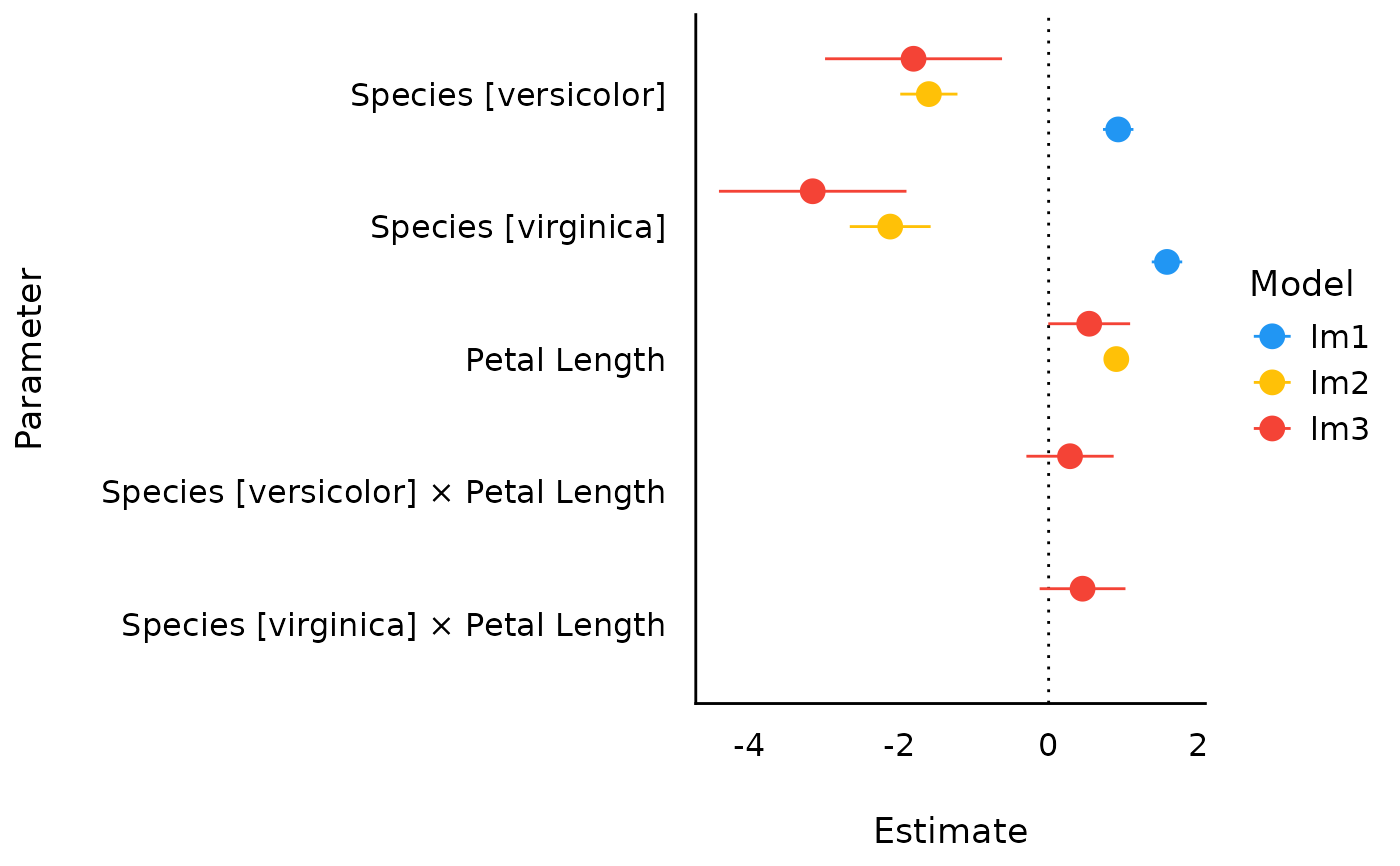The plot() method for the parameters::compare_parameters() function.

## Usage

# S3 method for see_compare_parameters
plot(
x,
show_intercept = FALSE,
size_point = 0.8,
size_text = NA,
dodge_position = 0.8,
sort = NULL,
n_columns = NULL,
show_labels = FALSE,
...
)

## Arguments

x

An object.

show_intercept

Logical, if TRUE, the intercept-parameter is included in the plot. By default, it is hidden because in many cases the intercept-parameter has a posterior distribution on a very different location, so density curves of posterior distributions for other parameters are hardly visible.

size_point

Numeric specifying size of point-geoms.

size_text

Numeric value specifying size of text labels.

dodge_position

Numeric value specifying the amount of "dodging" (spacing) between geoms.

sort

The behavior of this argument depends on the plotting contexts.

• Plotting model parameters: If NULL, coefficients are plotted in the order as they appear in the summary. Setting sort = "ascending" or sort = "descending" sorts coefficients in ascending or descending order, respectively. Setting sort = TRUE is the same as sort = "ascending".

• Plotting Bayes factors: Sort pie-slices by posterior probability (descending)?

n_columns

For models with multiple components (like fixed and random, count and zero-inflated), defines the number of columns for the panel-layout. If NULL, a single, integrated plot is shown.

show_labels

Logical. If TRUE, text labels are displayed.

...

Arguments passed to or from other methods.

## Value

A ggplot2-object.

## Examples

if (require("insight") &&
require("parameters") &&
packageVersion("insight") >= "0.13.0") {
data(iris)
lm1 <- lm(Sepal.Length ~ Species, data = iris)
lm2 <- lm(Sepal.Length ~ Species + Petal.Length, data = iris)
lm3 <- lm(Sepal.Length ~ Species * Petal.Length, data = iris)
result <- compare_parameters(lm1, lm2, lm3)
plot(result)
}